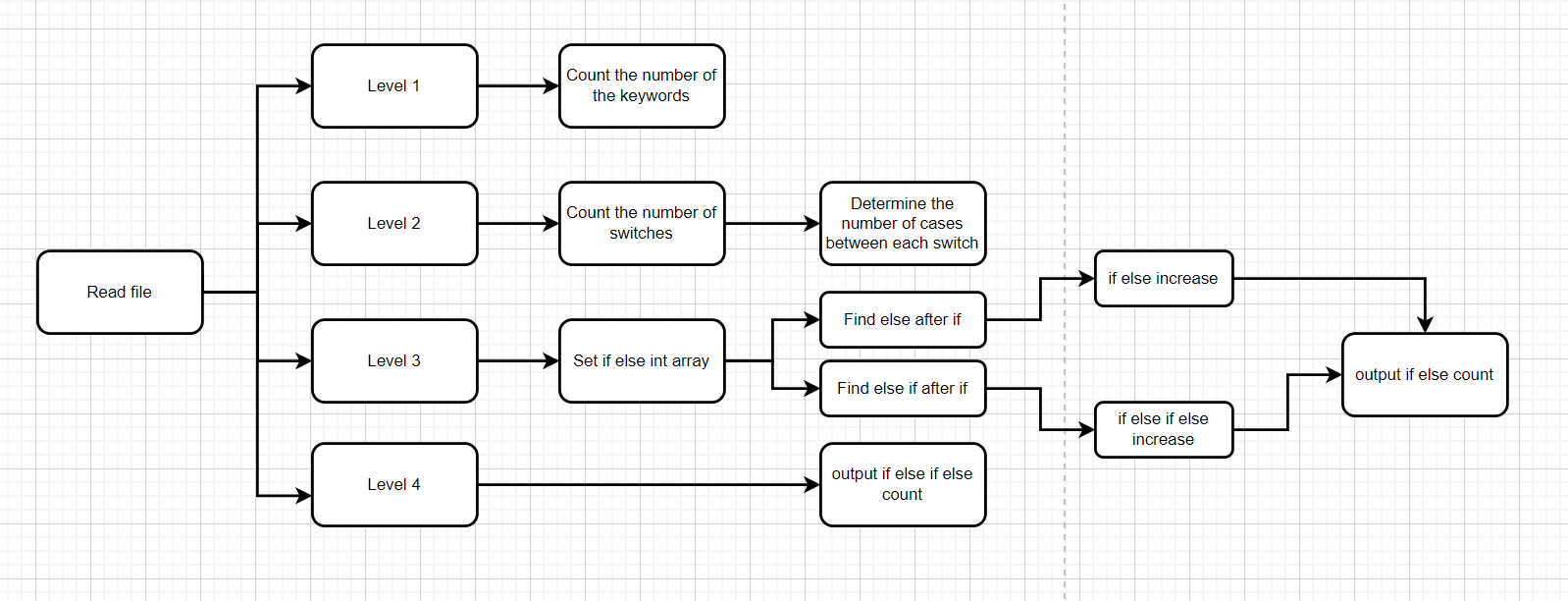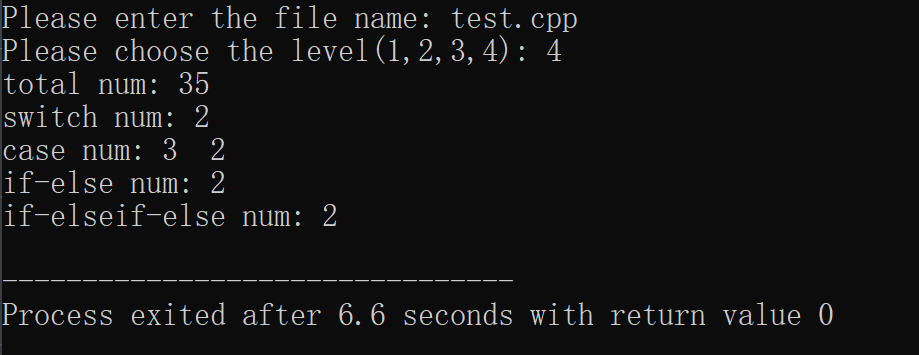# EE308-Lab1_2

The Link of Requirement of This Assignmenthttps://bbs.csdn.net/topics/608734907
The Aim of This AssignmentGitHub Using & Keywords Capturing
MU STU ID and FZU STU ID20121920_832002111
github :https://github.com/xxxtfish/xxxfish

PSP

Personal Software Process StagesESTIMATE TIME/MINUTESREAL TIME（MINUTE）
Planning4030
Estimate55
Development5055
Analysis4530
Design Spec1510
Design Review55
Coding Standard2015
Design6065
Coding200215
Code Review5045
Test2015
Reporting--
Test Report1510
Size Measurement1010
Postmortem&Process Improvement Plan2020
Total555530

Problem-solving idea
In the two years of university learning experience, although we have learned java, C and C++, personally, I am better at using C++ for programming, so my solution is to use C++ for code writing.

Design and implementation processCode

Main code

``````#include<iostream>
#include<fstream>
#include<cstdlib>
#include<iomanip>
#include<vector>
#include<algorithm>
using namespace std;
int main()
{
string test;
vector <string> nums;
string filename;
string KW = {"auto", "break", "case", "char", "const", "continue",
"default", "do", "double", "else", "enum", "extern", "float",
"for", "foto", "if", "int", "long", "main", "register", "return", "short",
"signed", "sizeof", "static", "struct", "switch", "typeof", "union",
"unsigned", "void", "volatile", "while"};
string ifelse_KW = {"else if","if","else"};
int KW_count = 0;
int switch_count = 0;
int case_count = 0;
int ifelse_KW_count = {0};
vector<int> casenum;
vector<string> ifelse;
int ifelse_count = 0;
int ifelseif_count = 0;
ifstream inFile;
cout<<"Please enter the file name: ";
cin>>filename;
int level;
cin>>level;
inFile.open(filename.c_str());
if(inFile.fail())
{
cout<<"The file was not successfully open!"<<endl;
exit(1);
}
string buf;
``````

Level 1

``````while (getline(inFile,buf))
{
int i = 1, j = 1;
while(i < int(buf.size()) && j < int(buf.size()))
{
if (isalpha(buf[i]))
{
if (!isalpha(buf[j] ))
{
string s = buf.substr(i, j-i);
nums.push_back(s);//The vector () function adds a new element to the end of a vector, one element after the current last element
for (int k = 0; k < 33; k++) {
if (s == KW[k]) {
KW_count += 1;
}
}
i = j+1;
j = i;
}
else
j++;
}
else{
i++;
j = i;
}
}
}
if(level >= 1)
cout<<"total num: "<<KW_count<<endl;

``````

Level 2

``````if(level > 1)
{
for(int i = 0;i < int(nums.size());i++)
{
if(nums[i] == "switch")
{
switch_count += 1;

for(int j = i+1;j < int(nums.size());j++)
{

if (nums[j] == "case")
case_count += 1;
else if(nums[j] == "switch" )
{
i=j-1;
casenum.push_back(case_count);
case_count = 0;
break;
}
else if(j == int(nums.size()-1))
{
i = j;
casenum.push_back(case_count);
break;
}
}
}
}
cout<<"switch num: "<<switch_count<<endl;
cout<<"case num: ";
for(int i = 0;i < int(casenum.size());i++)
{
cout<<casenum[i]<<"  ";
}
cout<<endl;
inFile.close();
}

``````

Level 3 and level 4

``````if(level>2)
{
inFile.open(filename.c_str());
if(inFile.fail())
{
cout<<"The file was not successfully open!";
exit(1);
}
while (getline(inFile,buf))
{
for(int i = 0;i < 3;i++)
{
if(int(buf.find(ifelse_KW[i]) != -1))
ifelse_KW_count[i] = 1;
else
ifelse_KW_count[i] = 0;
}
if(ifelse_KW_count == 1 && ifelse_KW_count == 1)
{
ifelse_KW_count=1;
ifelse_KW_count=0;
ifelse_KW_count=0;
}
for(int i = 0;i < 3;i++)
{
if(ifelse_KW_count[i] == 1)
ifelse.push_back(ifelse_KW[i]);
}
}
for(int i=0;i<int(ifelse.size())-1;i++)
{
if(ifelse[i] == "if" && ifelse[i+1] == "else")
{
ifelse_count += 1;
}
if(ifelse[i] == "if" && ifelse[i+1] == "else")
{
ifelseif_count += 1;
}
}
if(level>2)
cout<<"if-else num: "<<ifelse_count<<endl;
if(level>3)
cout<<"if-elseif-else num: "<<ifelseif_count<<endl;
}
return 0;
}

``````

OutputSummarize
Through this experiment, I learned how to publish blog in CSDN. Although there are a lot of things that are not very thoughtful, for me, this is completely new territory, and it is a breakthrough to dare to try new areas. Secondly, I learned how to use GitHub and how to upload code to GitHub. Overall, this experiment has been very rewarding!🙂

...全文
96 回复 打赏 收藏 举报论坛系列-cncode论坛源代码【含图文文档教程+源码导入教程+操作界面截图】05、R语言之七种武器 quantmod02a.mp4
05、R语言之七种武器 quantmod02a.mp4具有抗肿胀和皮肤无痕功能的湿式粘附多功能水凝胶 用于水下电生理监测和通信的无皮接口 监测和通信.pdfqt串口的例程，不知道好不好
qt串口的例程，不知道好不好基于java的办公自动化系统设计与实现毕业设计(源代码+说明报告+外文翻译)

286